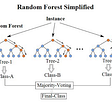# K-Fold Cross Validation

We often randomly split the dataset for better performance of model into train data and test data. The training data is used to train the model and for testing the model, we use test data which also offers us the evaluation of the model. Cross Validation is a widely used important technique which is preferably bought into action by scientists to measure the performance of machine learning model. The trouble with machine learning model is that no one knows how well a model performs or will perform until the tests are conducted on an independent dataset. Independent dataset is a dataset which is not used to train the machine learning model or we can say the train dataset. The accuracy of model varies as there is change in the random state of the split. To overcome this problem a cross validation technique is used to estimate the performance of the model. In this blog we are going to have a look on K-Fold Cross Validation.
K-Fold Cross Validation is a common type of cross validation that is widely used in machine learning.
The following steps are performed in K-Fold Cross Validation:
1. A dataset is split into a K number of sections or folds.
Let’s take a scenario where a data set is split into 6 folds.
2. After splitting the dataset, in first iteration, the first fold is used as testing data and remaining folds as training data.
3. In second iteration, the second fold is used as testing data and rest all as training data.
4. This process is repeated until each fold of all the 6 folds have been used as a testing data.
In the method of k-fold cross validation, all the entries in the original training dataset are used for both training as well as validating. Also, each entry is used for validation at least and at most once.

Applications of K-Fold Cross Validation
The k-fold cross validation is a technique which is used to compare the performances of different machine learning models on the same data set. For e.g. If there is a data set on which we have to apply several machine learning algorithms such as Regression, Random Forest, SVM (Support Vector Machine), Decision Tree, etc. To compare the performance of machine learning models on different algorithm and which algorithm we should choose to work upon, this technique will be of greater help.

Pros:
1. It helps us to make a better use of our data by using it in several ways.
2. We also can evaluate our model’s performance.
3. Reduces the risk of overfitting.
4. Better than randomly splitting data into train and test samples.

Cons:
1. Increase in training time, since with each iteration a model has to work from scratch.
2. Requires heavy computation as the required processing power is high.

--

--

--

## More from Analytics Vidhya

Analytics Vidhya is a community of Analytics and Data Science professionals. We are building the next-gen data science ecosystem https://www.analyticsvidhya.com

## Flower Classification using Transfer Learning and CNN (Step-by-Step)## Multi Layer Perceptron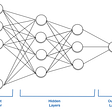## TensorFlow Model Optimization Toolkit — Post-Training Integer Quantization## Machine Learning for Executives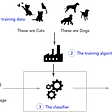## Research Papers Recommendation Engine with Sentence Transformer and Cosine Similarity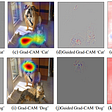## Exploring DVC for machine learning pipelines in research (Part 1)## Different Types of ML Bias and Ways to Detect it## Understanding and Implementing Classification Model Evaluation Method (Accuracy, Precision, Recall…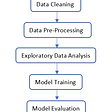## Fake News Detection using Machine Learning models## Active Learning in Classification — Query Strategies## Random Forest ML Algorithm